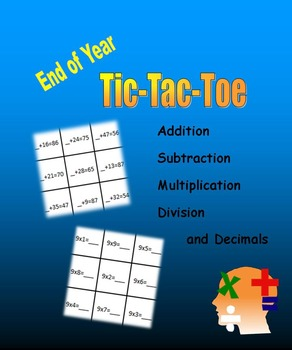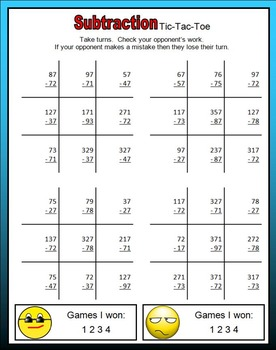# End of Year Tic-Tac-Toe (Addition, Subtraction, Multiplication, Division)2nd - 6th
Subjects
Standards
Resource Type
Formats Included
• PDF
• Compatible withActivities
Pages
56 pages
Compatible with Easel Activities
This resource is compatible with Easel by TpT, a suite of digital tools you can use to make any lesson interactive and device-ready. Customize this activity and assign it to students, all from Easel. Easel is free to use! Learn more.

#### Also included in

1. This bundle contains 180 printable multi-digit and one-digit multiplication printable or digital pages: world records, tic-tac-toe, spring season, word name, the 50 US states, and more. PRINT: I created a PDF version that you can download on your computer and print for students to use in class or at
\$10.00
\$19.99
Save \$9.99

### Description

42 printable pages combining Tic-Tac-Toe with addition, subtraction, multiplication and division:

• decimal addition (tenths, hundredths and thousandths)
• two-digit subtraction,
• three-digit subtraction,
• four-digit subtraction,
• decimal subtraction (tenths, hundredths and thousandths)
• one-digit multiplication
• two-digit multiplication
• three-digit multiplication
• four-digit multiplication
• one-digit division

Take a look at the Preview to sample pages in this packet. And if you would like more END OF YEAR activities, here are the others in this series:

End of Year Division, Squares & World Records

End of Year Writing, Words & Whales

End of Year Money, Measuring & Multiplication

End of Year Multiplication Games (Bingo, Spinner, Dice)

End of Year Problem-Solving (Brainteasers, Patterns, Sequence, Word Puzzles)

End of Year Tic-Tac-Toe (Addition, Subtraction, Multiplication, Division)

End of Year Awards & Certificates

And if you would like more on MULTIPLICATION or DIVISION, take a look at these:

Multiplication Flashcards

Multiplication & Division Memory Match Game

Product or Quotient: A Spinner Game

Multiplication: Sequence Game

Multiplication and Word Puzzles

Daily Multiplication with Dice

Multiplication Tic-Tac-Toe

Multiplication Game - Four-in-a-row

Spinner Multiplication Game

Idioms and One-digit Multiplication

Multiplication Chart - Fix the Errors

Multiplication Chart - Missing Numbers

Multiplication - Draw a picture - Write a story

Multiply and Divide within 100

One-digit Multiplication

Multi-digit Multiplication

Four Operations Math Challenge

Four Operations Math Challenge (2)

Monster Multiplication (Multiply within 100)

Presidential Multiplication

Multiplication & Place Value

Word Name: Multiplication and Division

Multiplication: Find the Errors

Multiplication Mastery: with a daily Word Puzzler and Geometry Challenge

Five-minute Multiplication Challenge (Math Warm-ups)

Multiplication - Find the multiples - Find the equations

Fractions and Multiplication

Division: The 50 States

Division: World Records

Division and the USA

First Day of School: Multiplication & Division Games

End of Year: Division, Squares and World Records

End of Year Tic-Tac-Toe: Addition, Subtraction, Multiplication, Division

And here are some fully-animated PowerPoint slideshows:

How to use a Multiplication Chart

55 Multiplication Facts in One Minute

Two-minute Multiplication

Multiplication from 1x1 to 10x10

Multiplication Facts in Five Minutes

Division Facts in Five Minutes

Division with Remainders

Multiplication Facts: Where is the Mistake?

Division within 100 PowerPoint BUNDLE

Total Pages
56 pages
Does not apply
Teaching Duration
N/A
Report this Resource to TpT
Reported resources will be reviewed by our team. Report this resource to let us know if this resource violates TpT’s content guidelines.

### Standards

to see state-specific standards (only available in the US).
Fluently multiply and divide within 100, using strategies such as the relationship between multiplication and division (e.g., knowing that 8 × 5 = 40, one knows 40 ÷ 5 = 8) or properties of operations. By the end of Grade 3, know from memory all products of two one-digit numbers.
Fluently multiply multi-digit whole numbers using the standard algorithm.
Find whole-number quotients and remainders with up to four-digit dividends and one-digit divisors, using strategies based on place value, the properties of operations, and/or the relationship between multiplication and division. Illustrate and explain the calculation by using equations, rectangular arrays, and/or area models.
Read and write multi-digit whole numbers using base-ten numerals, number names, and expanded form. Compare two multi-digit numbers based on meanings of the digits in each place, using >, =, and < symbols to record the results of comparisons.
Fluently add and subtract within 1000 using strategies and algorithms based on place value, properties of operations, and/or the relationship between addition and subtraction.